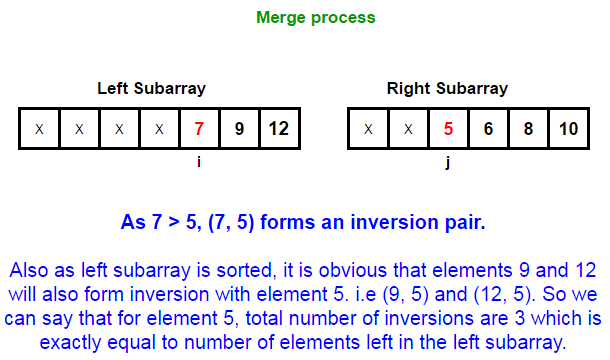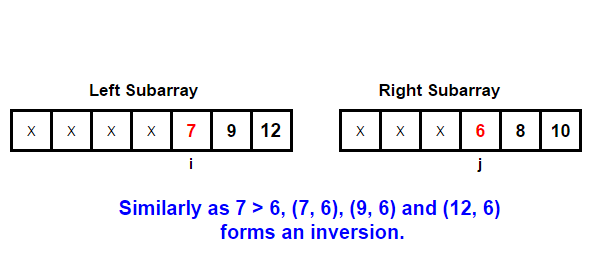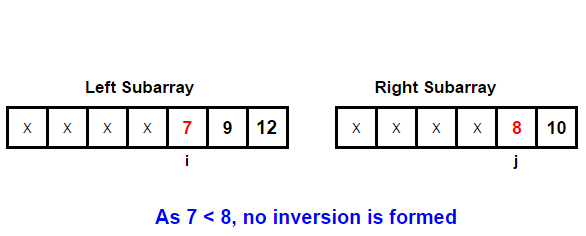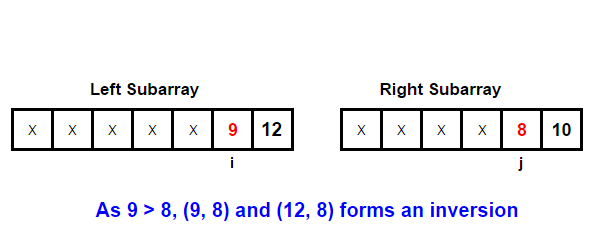# Inversion count of an array

Given an array, find the number of inversions of it. If (i < j) and (A[i] > A[j]) then the pair (i, j) is called an inversion of an array A. We need to count all such pairs in the array.

For example,

Input:  A[] = [1, 9, 6, 4, 5]
Output: Inversion count is 5

There are 5 inversions in the array – (9, 6), (9, 4), (9, 5), (6, 4), (6, 5)

##### Approach 1: Naive

Simple solution would be for each element of the array, we count all elements less than it to its right and add the count to output. The complexity of this solution is O(n2).

## Java

Output:

Inversion count is 5

##### Approach 2: (Using Merge Sort)

This is a classic problem that can be solved by Merge Sort Algorithm. Basically for each element of the array, we count all elements more than it to its left and add the count to output. This whole magic happens inside merge function of mergesort.

Let us consider two sub-arrays involved in merge process –Below’s C and Java program that demonstrates it:

## Java

Output:

Inversion count is 5

Time complexity of above solution is same as that of merge sort algorithm i.e. O(nlogn) and auxiliary space used by the program is O(n).(3 votes, average: 5.00 out of 5)Loading...

Please use our online compiler to post code in comments. To contribute, get in touch with us.
Like us? Please spread the word and help us grow. Happy coding 🙂Subscribe
Notify ofGuest

nice..Guest

Thank you so much!Guest
arandomguy

Please add another approach using binary indexed tree (BIT).